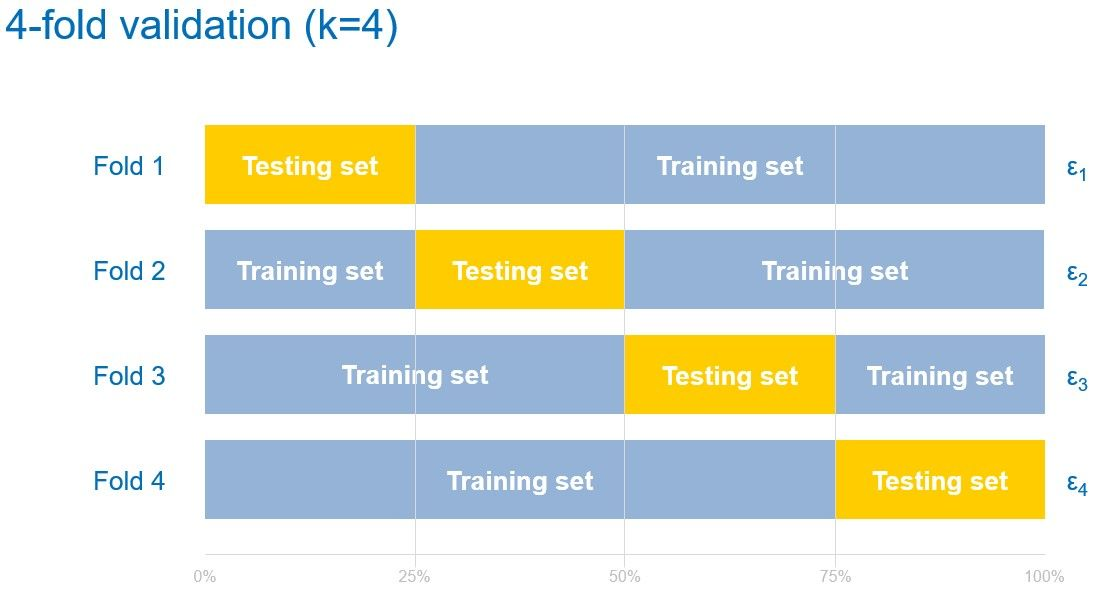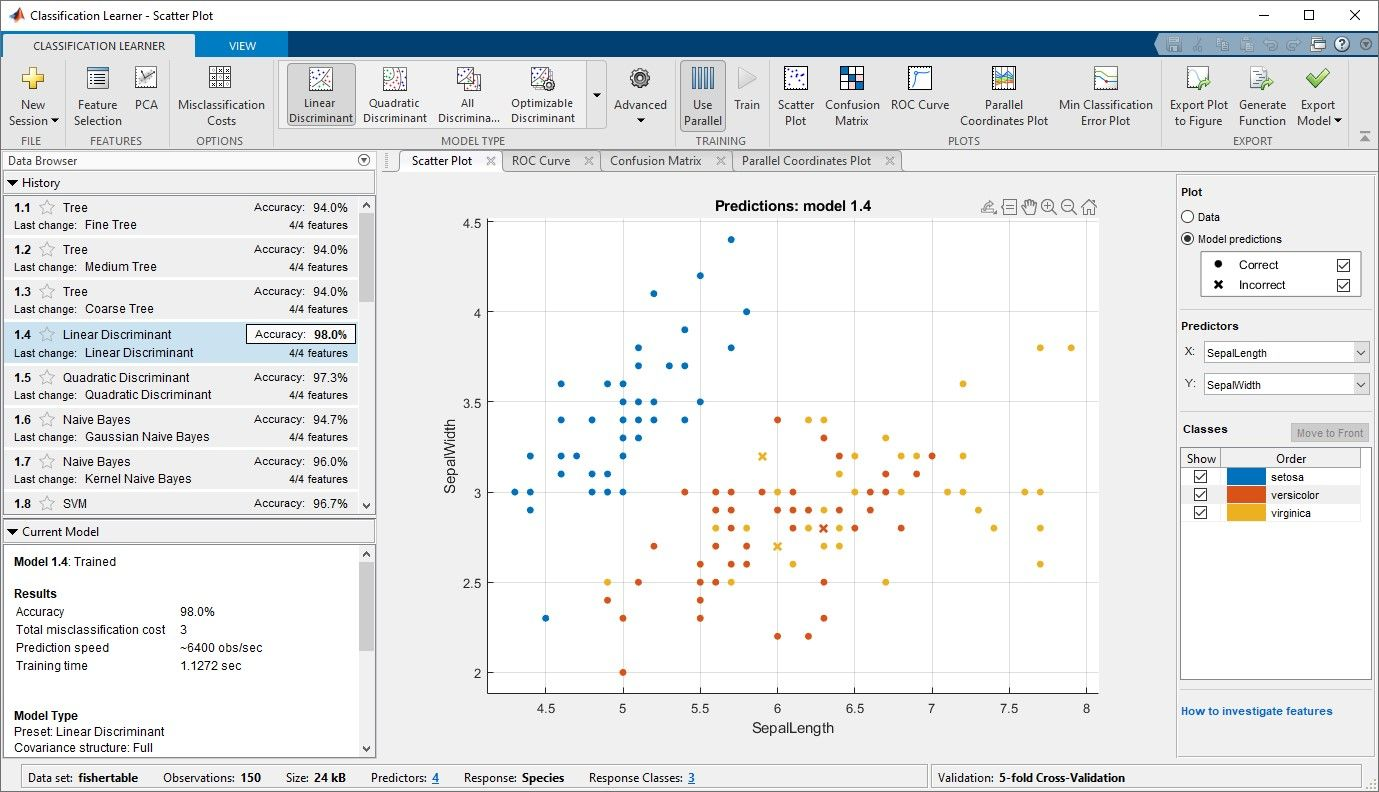# Cross-Validation

## Assess and improve predictive performance of models

Cross-validation is a model assessment technique used to evaluate a machine learning algorithm’s performance in making predictions on new datasets that it has not been trained on. This is done by partitioning the known dataset, using a subset to train the algorithm and the remaining data for testing.

Each round of cross-validation involves randomly partitioning the original dataset into a training set and a testing set. The training set is then used to train a supervised learning algorithm and the testing set is used to evaluate its performance. This process is repeated several times and the average cross-validation error is used as a performance indicator.

### Why Is Cross-Validation Important?

When training a model, it is important not to overfit or underfit it with algorithms that are too complex or too simple. Your choice of training set and test set are critical in reducing this risk. However, dividing the dataset to maximize both learning and validity of test results is difficult. This is where cross-validation comes into practice. Cross-validation offers several techniques that split the data differently, to find the best algorithm for the model.

Cross-validation also helps with choosing the best performing model by calculating the error using the testing dataset, which has not been used to train. The testing dataset helps calculate the accuracy of the model and how it will generalize with future data.

### Common Cross-Validation Techniques

Many techniques are available for cross-validation. Among the most common are:

• k-fold: Partitions data into k randomly chosen subsets (or folds) of roughly equal size. One subset is used to validate the model trained using the remaining subsets. This process is repeated k times such that each subset is used exactly once for validation. The average error across all k partitions is reported as ε. This is one of the most popular techniques for cross-validation but can take a long time to execute because the model needs to be trained repeatedly. The image below illustrates the process.• Holdout: Partitions data randomly into exactly two subsets of specified ratio for training and validation. This method performs training and testing only once, which cuts execution time on large sets, but interpret the reported error with caution on small data sets.
• Leaveout: Partitions data using the k-fold approach where k is equal to the total number of observations in the data and all data will be used once as a test set. Also known as leave-one-out cross-validation (LOOCV).
• Repeated random sub-sampling: Creates multiple random partitions of data to use as training set and testing set using the Monte Carlo methodology and aggregates results over all the runs. This technique has a similar idea as the k-fold but each test set is chosen independently, which means some data points might be used for testing more than once.
• Stratify: Partitions data such that both training and test sets have roughly the same class proportions in the response or target.
• Resubstitution: Does not partition the data and all data is used for training the model. The error is evaluated by comparing the outcome against actual values. This approach often produces overly optimistic estimates for performance and should be avoided if there is sufficient data.

Cross-validation can be a computationally intensive operation since training and validation is done several times. However, it is a critical step in model development to reduce the risk of overfitting or underfitting a model. Because each partition set is independent, you can perform this analysis in parallel to speed up the process. For larger datasets, techniques like holdout or resubstitution are recommended, while others are better suited for smaller datasets such as k-fold and repeated random sub-sampling.

### Cross-Validation with MATLAB

MATLAB® supports cross-validation and machine learning. You can use some of these cross-validation techniques with the Classification Learner App (4:34) and the Regression Learner App (3:42).Classification Learner app for training, validating, and tuning classification models. The history list shows various classifier types.Regression Learner app for training, validating, and tuning regression models. The history list includes various regression model types.

To speed computationally intensive operations, you can perform parallel computations on multicore computers, GPUs, and clusters with Parallel Computing Toolbox™.

For more information on using cross-validation with machine learning problems, see Statistics and Machine Learning Toolbox™ and Deep Learning Toolbox™ for use with MATLAB.

See also: Statistics and Machine Learning Toolbox, machine learning, supervised learning, feature selection, regularization, linear model, ROC Curve